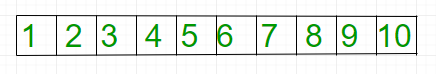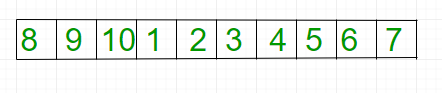Open In App

# Python3 Program for Reversal algorithm for right rotation of an array

Given an array, right rotate it by k elements.After K=3 rotationExamples:

```Input: arr[] = {1, 2, 3, 4, 5,
6, 7, 8, 9, 10}
k = 3
Output: 8 9 10 1 2 3 4 5 6 7

Input: arr[] = {121, 232, 33, 43 ,5}
k = 2
Output: 43 5 121 232 33```

Note : In the below solution, k is assumed to be smaller than or equal to n. We can easily modify the solutions to handle larger k values by doing k = k % n
Algorithm:

```rotate(arr[], d, n)
reverseArray(arr[], 0, n-1) ;
reverse(arr[], 0, d-1);
reverse(arr[], d, n-1);```

Below is the implementation of above approach:

## Python3

 `# Python3 program for right rotation of``# an array (Reversal Algorithm)`  `# Function to reverse arr``# from index start to end``def` `reverseArray( arr, start, end):``    ` `    ``while` `(start < end):``        ` `        ``arr[start], arr[end] ``=` `arr[end], arr[start]``        ``start ``=` `start ``+` `1``        ``end ``=` `end ``-` `1``    `  `# Function to right rotate arr``# of size n by d``def` `rightRotate( arr, d, n):``    ` `    ``reverseArray(arr, ``0``, n ``-` `1``);``    ``reverseArray(arr, ``0``, d ``-` `1``);``    ``reverseArray(arr, d, n ``-` `1``);`  `# function to print an array``def` `printArray( arr, size):``    ``for` `i ``in` `range``(``0``, size):``        ``print` `(arr[i], end ``=` `' '``)`  `# Driver code``arr ``=` `[``1``, ``2``, ``3``, ``4``, ``5``, ``6``, ``7``, ``8``, ``9``, ``10``]``n ``=` `len``(arr)``k ``=` `3``    ` `# Function call``rightRotate(arr, k, n)``printArray(arr, n)`  `# This article is contributed``# by saloni1297`

Output:

`8 9 10 1 2 3 4 5 6 7 `

Time Complexity: O(N)

Auxiliary Space: O(1)

Please refer complete article on Reversal algorithm for right rotation of an array for more details!